# SBI PO Prelims Reasoning Questions 2019 (Day-43)

Dear Aspirants, Our IBPS Guide team is providing new series of Reasoning Questions for SBI PO 2019 so the aspirants can practice it on a daily basis. These questions are framed by our skilled experts after understanding your needs thoroughly. Aspirants can practice these new series questions daily to familiarize with the exact exam pattern and make your preparation effective.

[WpProQuiz 6325]

### Click Here for SBI PO Pre 2019 High-Quality Mocks Exactly on SBI Standard

Directions (1-5): Study the following information carefully and answer the below questions.

Certain numbers of people are sitting in a row facing south. D sits to the immediate right of A.Q sits exactly between D and R. P sits at the left end of the row. Number of people sitting to the right of D is one less than the number of people to the left of D. N and R are immediate neighbors. There are not more than thirteen and less than five seats in the row. Not more than two people are sitting together. N sits at one of the extreme ends. S is an immediate neighbor of P. C is sitting at one of the positions.

1) How many seats are there in the row?

a) 8

b) 9

c) 13

d) 10

e) 12

2) How many people are sitting in the row?

a) 11

b) 8

c) 9

d) 10

e) 13

3) Who among the following sits exactly between P and D?

a) C

b) Q

c) S

d) R

e) A

4) Four of the following five are alike in a certain way and thus form a group. Who among the following does not belong to the group?

a) N

b) R

c) A

d) Q

e) P

5) How many seats are there between D and C?

a) 1

b) 3

c) 2

d) None

e) None of these

Directions (6-10): Study the following information carefully considering the below given series and answer the below questions.

567    987    243    559    727

6) If the above series is arranged in descending order then which among the following will be the third digit from the right end of the second number from the left end?

a) 3

b) 7

c) 9

d) 5

e) 2

7) What is the difference between the third digit of the number which is second from the left end and the first digit of the number that is first from the right end as given in the series?

a) 2

b) 5

c) 4

d) 1

e) 0

8) If ‘1’ is added to all the even digits and ‘2’ is subtracted from all odd digits then how many 3’s can be found in the above series?

a) 7

b) 2

c) 5

d) 6

e) 4

9) If all the even digits are removed then what is the sum of first three digits from the right end and last two digits from the left end as in the given series?

a) 35

b) 27

c) 33

d) 38

e) 42

10) What is the minimum difference between any two numbers in the above given series?

a) 11

b) 9

c) 7

d) 8

e) 0

Directions (1–5):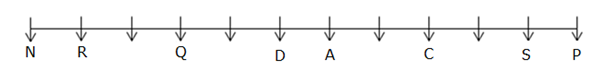• There are not more than thirteen and less than five seats in the row. Number of people sitting to the right of D is one less than the number of people to the left of D.
• On calculating seating positions using the above given conditions we get four possible cases: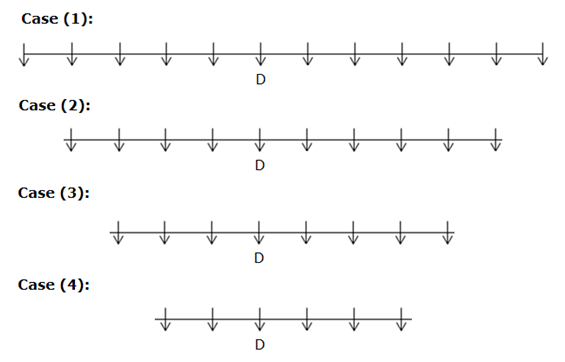• P sits at the left end of the row. N sits at one of the extreme ends. N and R are immediate neighbors.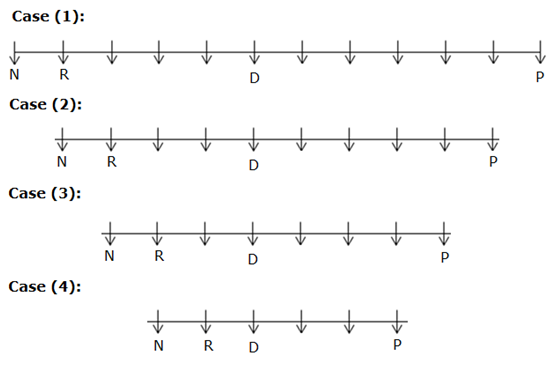• Q sits exactly between D and R. Not more than two people are sitting together.
• Here Case (3) and Case (4) gets eliminated as not more than two people are sitting together.
• Case (2) also gets eliminated as Q sits exactly between D and R.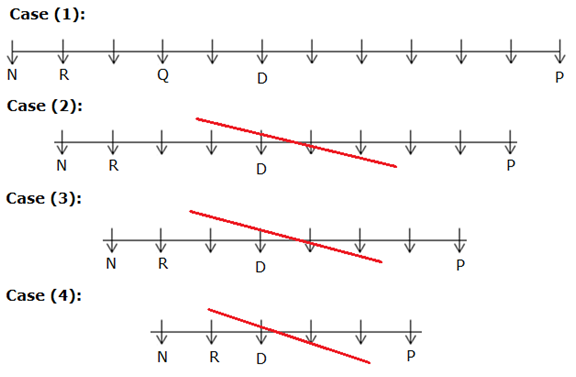• D sits to the immediate right of A. S is an immediate neighbor of P.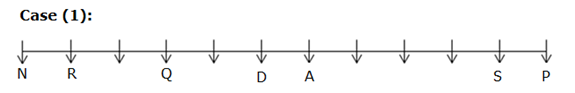• C is sitting at one of the positions. So the final arrangement is,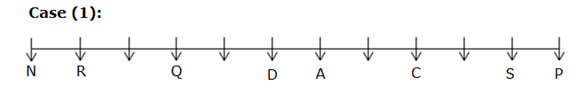Directions (6–10):

567    987    243    559    727

After rearrangement,

987    727   567    559    243

Third digit from the right end of the second number from the left end is 7.

Third digit of the number which is second from the left is 7.

First digit of the number that is first from the right end is 7.

So the difference is 0.

375    795    351    337    535

‘1’ is added to all the even numbers and ‘2’ is subtracted from all odd numbers, then 5 3’s can be found.

57      97      3        559    77

Here, all the even numbers are removed.

Sum of first three digits from the right end and last two digits from the left end is,

7+7+9+5+7 = 35

Minimum difference between the numbers is,

567 – 559 = 8

Related Articles :

SBI PO Prelims Reasoning Questions 2019 (Day-42)

SBI PO Prelims Reasoning Questions 2019 (Day-41)